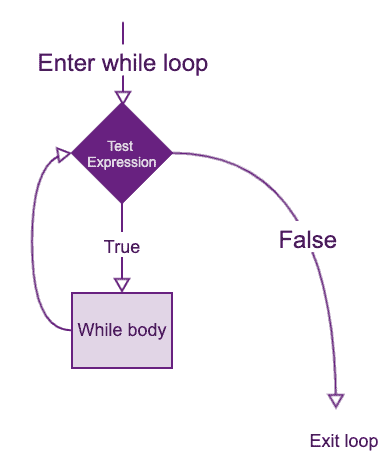# R While Loop: How to Use While Loop in R

The While loop executes the same code continuously until the stop condition returns TRUE. A loop is a statement that keeps running until a condition becomes FALSE

## R While loop

The while loop in R executes continuously until the loop breaks or met the condition that ends the loop. The while loop control statement will run a statement or a set of statements repeatedly unless the given condition becomes FALSE or the stop condition is met.

### Syntax

``````while (test_expression)
{
statement
}``````

Here, the test_expression is evaluated, and the loop’s body is entered if the result is TRUE.

The statements inside the loop are executed, and the flow returns to re-assess the test_expression.

This is repeated each time until test_expression assesses to FALSE, in which case, the loop exits.

### Flow chart of while loop### Example of while loop in R

Let’s see the example.

``````# Create a variable with value 1
start <- 1

# Create the loop
while (start <= 10) {

# See which we are
cat("This is loop number", start, "\n")

# Add 1 to the variable start after each loop
start <- start + 1
}``````

#### Output

``````This is loop number 1
This is loop number 2
This is loop number 3
This is loop number 4
This is loop number 5
This is loop number 6
This is loop number 7
This is loop number 8
This is loop number 9
This is loop number 10``````

In this example, the start is initially initialized to 1.

Here, the test_expression start <= 10, which evaluates TRUE since 1 is less than 10. So, the body of the loop is entered, and the start is printed and incremented.

Incrementing i is important as this will eventually meet the exit condition. Failing to do so will result in an infinite loop.

In the next iteration, the value of start is 2, and the loop continues.

This will continue until the start takes the value of 10. The condition 11 <= 10 will give FALSE, and the while loop finally exits.

## Calculate the factorial using while loop

To calculate the factorial of a number in R, use the while loop.

``````n <- 6

# Assigning the factorial and iteration variable to 1
factorial <- 1
i <- 1

# Start the while loop
while (i <= n) {

# Multiplying the factorial variable with an iteration variable
factorial <- factorial * i

# Increment an iteration variable
i <- i + 1
}

# Show the factorial
print(factorial)``````

#### Output

`` 720``

Here, in this program, we have used three variables.

1. loop
2. factorial
3. i

The loop variable defines the total number of iterations. The value of the loop variable is 6, which means the while loop will be executed 6 times.

The initial value of the factorial and i variable is 1.

The i variable will be used for iterating over the loop, and the factorial will be used for calculating the factorial. In each iteration of the loop, the condition is checked, i.e., i should be less than or equal to 6, and after that factorial is multiplied with the value of i, then i is incremented.

When i become 7, the loop is terminated, and the factorial of 6, i.e., 720, is displayed beyond the scope of the loop.

That is it for this tutorial.